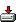3D Calculator

This calculator can be used for

- Vector Calculation
Subtract, multiply (dot or cross), find magnitude, unit vector, angle and scalar triple products of vectors.

- Point, Line, Plane
Find equation of line, plane in two point form, parametric form or volume and surface area of sphere.

- Coordinate System
Convert Cartesian to spherical or cylindrical coordinate or vice versa.

- Matrices
Add, subtract, multiply the matrices, find determinants or solutions of Ax=b for 3×3 matrices.

- Complex Numbers
Add, subtract, multiply, divide complex numbers, find exponent, log, trigonometric and hyperbolic trigonometric values of complex numbers.

Homepage: http://www.greuer.de/calc3D.html•Direct link
• software/3d_calculator